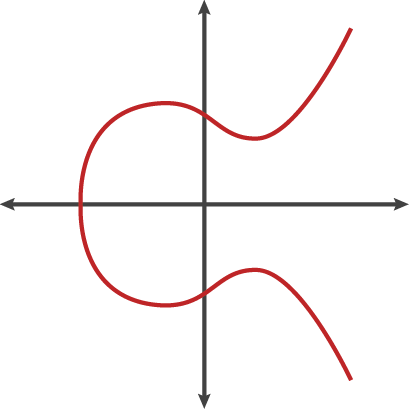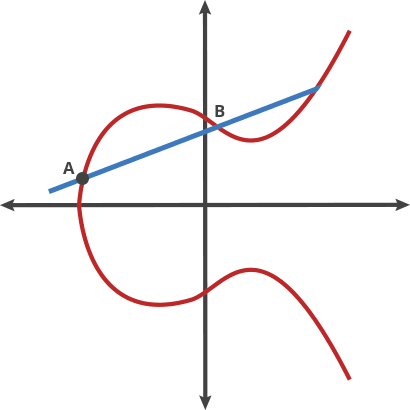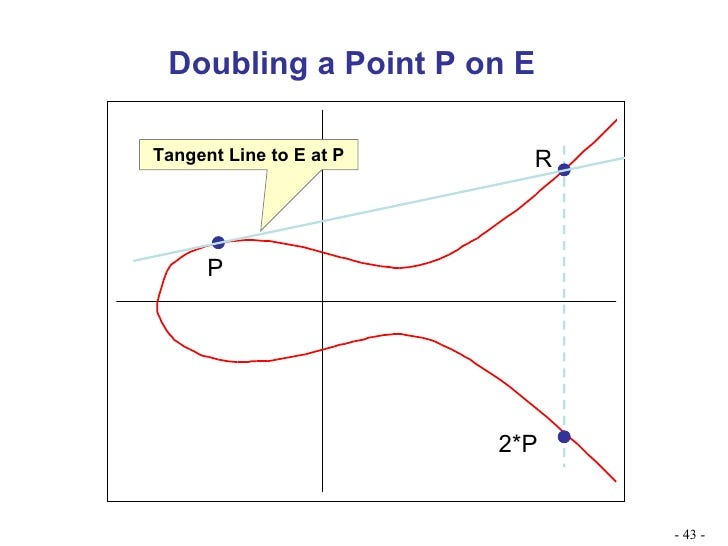# Understanding Schnorr Signatures

##### 2019-03-20T16:49:00.000ZHonest Cash

On May 15th of this year Bitcoin Cash will be activating a hardfork which introduces Schnorr signatures. This post is intended to be a very high level overview of the math behind Schnorr signatures (or at least the BCH variant) for people who may not understand elliptic curve cryptography. We'll start with the basics.

## Generating a key pair

In Bitcoin we use a curve with the equation:

``````y² = x³ + 7
``````When plotted on a graph the curve ends up looking something like above.

From here we will define a way to "add" two points on the curve together. I put "add" in quotes because this isn't the same addition that you would do on ordinary (scalar) numbers. Ordinary addition isn't really something you can do with two points. The term "add" is also somewhat made up. It could have been called "banana" because it's somewhat arbitrary. But we will see later on there are some parallels between this elliptic curve "add" operation and the addition we are used to doing with ordinary numbers.

To "add" two points you draw a line between the two points, find where that line intersects with the curve at a third point, then reflect that third point over the x-axis. It ends up kind of looking like a weird game of billiards.The above image shows:

``````A + B = C
A + C = D
A + D = E
``````

Keep in mind we're dealing with points here. The "sum" of two points is also a point on the curve.

Finally we will define a second operation which we will call "double". This does what it sounds like ―it adds a number to itself:

``````A + A = 2A
``````

To perform the "double" you take the tangent of the point, find where the line intersects with curve, then reflect that point over the x-axis.Now that we have "doubling" we can also do multiplication. Multiplication is just repeated addition after all. So if you wanted to find the point at `9A` you could do an initial "double" to get to `2A` plus seven add operations.

``````2A + A + A + A + A + A + A + A = 9A
``````

This works but notice there's a shortcut. Doing it the above way took us eight operations ― one double plus seven adds. But we could have also done three doubles plus one add to get to `9A` in only four operations.

``````2A -> 4A -> 8A + A = 9A
``````

Using this shortcut we can actually multiply points by extremely large numbers since we only need to perform a logarithmic number of operations to find the point.

But notice, that while we have a really nice shortcut which makes multiplication very fast, we don't have any division operation. If I give you a point on the curve that is the product of multiplying A * N where, N is a very large number, you wont have any way to divide the point to give you N.

Thus we have a nice trap door function which we can use to create a key pair.

The secp256k1 curve parameters defines a generator point (G) which we use as the starting point for our operations. Our "private key" (x) is just a very large random number. To get to the "public key" we multiply the private key (using the ECMultiply operation) by the generator point (G). The resulting point on the curve is our public key.

``````x * G = P
``````

Notice again our private key is just an ordinary (scalar) number while our public key is a point on the curve. We can use the doubling shortcut we described above to calculate the public key (the point) that corresponds to our private key, but someone in possession of our public key cannot divide the point by G to get our private key since there is no divide operation that can be performed.

That's it. It's actually fairly simple from a high level. Of course in practice there's more to it. We have to do all these operations modulo a large prime number and there are some other considerations but if you're interested in learning more you can take a deep dive into elliptic curve cryptography.

There's an interesting property of these key pairs in that if you add (or multiply) a number to both the private key and public keys you get a new key pair that is still valid. That is, the new public key corresponds to the new private key. For example, suppose we have a private key of `9` and public key of `9G` and we add four to both:

``````9 * G = point(9G)
+ 4           + 4G
-------------------
13 * G = point(13G)
``````

Notice since the private key is a scalar value we can just add four to it like normal. But since the public key is a point we have to add another point to it, so we add `4 * G`. The resulting key pair is still perfectly valid. The same trick works for multiplication. We're going to need this property when signing and verifying Schnorr signatures.

## Signing with Schnorr

So we have our private key `x` and our public key `P` and we want to create a Schnorr signature. Let's go through the following steps.

Step 1: Create a new, ephemeral key pair. This ephemeral private key must be random. If it isn't there's a chance your private key could be compromised. In BCH we actually suggest using a deterministic algorithm to derive the ephemeral private key from the long lived private key and the message to be signed using a modified version of RFC6979.

``````R = k * G
``````

In the above equation `R` is the ephemeral public key and `k` is the ephemeral private key.

Step 2: Hash the message. We could just hash the message outright but in our scheme we concatenate the message with long lived public key `P` and the ephemeral public key `R` to ensure the hash in unique.

``````e = SHA256(R || P || m)
``````

Above, `e` is our hash and `m` is the message to be signed.

Step 3: Compute the `s` value:

``````s = k + e * x
``````

Note that `k`, `e` and `x` are all scalar values so we are just doing regular addition and multiplication and `s` is also a scalar.

Finally the signature that we will send to the verifier consists of point `R` (the ephemeral public key) and scalar `s`. Technically since `R` is a point we only need to send the x-coordinate of `R` to the verifier so our signature ends up being the serialization of `(32 byte R.x) || (32 byte s)`. For a total of 64 bytes.

## Verifying the signature

The verifier now has `P` (the signers public key), `m` (the message), `R` (the ephemeral public key) and `s`.

We now have enough information to calculate the same `e` value (the hash) that the signer calculated.

``````e = SHA256(R || P || m)
``````

The trick now is to independently calculate the `R` point and verify that the `R` point we calculated matches the `R` point that the signer sent us. If it matches, we have a valid signature. If it doesn't, the signature is invalid.

Let's take a look at the equation the signer used to calculate `s`.

``````s = k + e * x
``````

Using a little algebra we can rewrite this to solve for `k` (the private key for `R`).

``````k = s - e * x
``````

Since we are trying to independently calculate `R`, we should be able to do so if we are able to find the public keys that correspond to `s` and `x` (we already calculated `e` in the previous step).

We already know the public key that corresponds to `x` since this is the signers long lived public key `P` which we received from the signer.

Since `s` is just a scalar value we can treat it as if it was a private key. Private keys are just large numbers after all. To get the public key for `s` we just multiply `s` by the generator point.

So the equation to find `R` now becomes:

``````R = s * G - e * P
``````

We've substituted the public keys corresponding to `k`, `s` and `x` into the original equation.

We are now in a position to verify that our calculated point `R` equals the point `R` that was given to us as part of the signature.

So that's it. Pretty easy to understand once you get rid of the jargon. Hope this helps you understand the May 15th Bitcoin Cash hardfork better.

## RE: Understanding Schnorr Signatures

by @siddartha

Wow, that's pretty detailed. And you say it is just a high level overview!

I didn't knew that cryptography, private keys etc. had a relation to graphs. That's new for me.

## RE: Understanding Schnorr Signatures

by @Telesfor

Thanks for the explanation, I think I understood Schnorr Signatures a bit better now.